# PrimerDigital

## Melting temperature calculation

The melting temperature (Tm) is defined as the temperature at which half the DNA strands are in the double-helical state and half are in the "random-coil" state.
The Tm for short oligonucleotides with normal or degenerate (mixed) nucleotide combinations are calculated in the default setting using nearest neighbour thermodynamic parameters.
The Tm is calculated using a formula based on nearest neighbour thermodynamic theory with unified dS, dH and dG parameters (Allawi and SantaLucia, 1997):where dH is enthalpy for helix formation, dS is entropy for helix formation, R is molar gas constant (1.987cal/K mol), c is the nucleic acid molar concentration (250 pM), [K+] is salt molar concentration (default value is 50 mM).

The Tm for mixed bases is calculated by averaging nearest neighbour thermodynamic parameters - enthalpy and entropy values - at each mixed site; extinction coefficient is similarly predicted by averaging nearest neighbour values at mixed sites.

The mismatched pairs can be taken into account, the parameters being provided for DNA/DNA duplexes and the dangling ends that are unmatched terminal nucleotides being provided for DNA/DNA duplexes. The melting temperature for primer (probe) self or cross dimers and for in silico PCR experiments with oligonucleotides having mismatches to the target is calculated using values for the thermodynamic parameters for a nucleic acid duplex.

For non-thermodynamic Tm calculation using simple formulae; the Wallace-Ikatura rule is often used as a rule of thumb when primer Tm is to be estimated at the bench.
However, the formula was originally applied to the hybridisation of probes in 1M NaCl and is an estimate of the melting temperature for short oligonucleotides: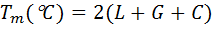for longer 14 bases: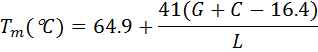where G+C - the number of G's and C's and L - primer length.

Marmur and Doty originally established a formula to correlate G+C content to the Tm of long duplexes at a given ionic strength.
Chester and Marshak added a term to account for DNA strand length (L) to estimate primer Tm: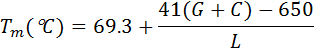The Marmur–Schildkraut–Doty equation also accounts for ionic strength with a term for the K+ concentration. Another modification is that of Wetmur: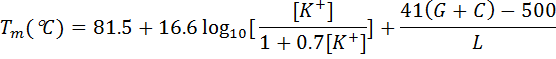von Ahsen et al. (2001) formulae: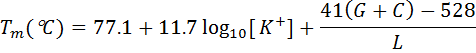Two equations above assume that the stabilizing effects of cations are the same on all base pairs. The melting temperature of the PCR product calculated using the formula: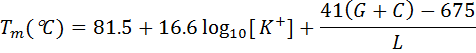﻿